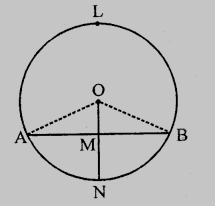# Prove that the line joining the mid-point of a chord to the centre of the circle passes through the mid-point of the corresponding minor arc.

To do:

We have to prove that the line joining the mid-point of a chord to the centre of the circle passes through the mid-point of the corresponding minor arc.

Solution:

Let a circle with centre $O$ and a chord $AB$, $M$ be the mid point of $AB$ and $OM$ is joined and produced to meet the minor arc $AB$ at $N$.Join $OA$ and $OB$

$M$ is the mid point of $AB$

This implies,

$OM \perp AB$

In $\triangle OAM$ and $\triangle OBM$,

$OA = OB$               (Radii of the circle)

$OM = OM$            (common)

$AM = BM$           ($M$ is the mid point of $AB$)

Therefore, by SSS axiom,

$\triangle OAM = \triangle OBM$

This implies,

$\angle AOM = \angle BOM$        (CPCT)

$\angle AOM = \angle BOM$

These are the angles at the centre made by arcs $AN$ and $BN$.

Thetrefore,

$Arc\ AN = Arc\ BN$

Hence, $N$ divides the arc in two equal parts.

Updated on: 10-Oct-2022

46 Views